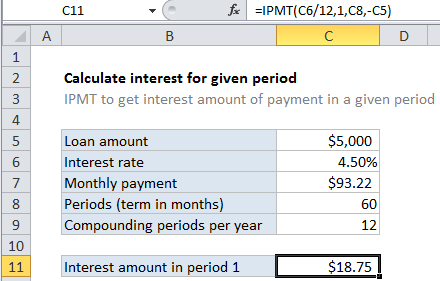## Excel Office

Excel How Tos, Tutorials, Tips & Tricks, Shortcuts

# Calculate interest for given period in Excel

To calculate the interest portion of a loan payment in a given period, you can use the IPMT function.

## Formula

`=IPMT(rate,period,periods,-loan)`## Explanation

In the example shown, the formula in C10 is:

`=IPMT(C6/12,1,C8,-C5)`

### How this formula works

For this example, we want to calculate the interest portion for payment 1 of a 5-year loan of \$5,000 with an interest rate of 4.5%. To do this, we set up PPMT like this:

Worked Example:   IPMT function: Description, Usage, Syntax, Examples and Explanation

rate – The interest rate per period. We divide the value in C6 by 12 since 4.5% represents annual interest:

`=C6/12`

pv – The present value, or total value of all payments now. In the case of a loan, this is input as a negative value by adding a negative sign in front of C5 to supply -5000.

Worked Example:   IPMT function: Description, Usage, Syntax, Examples and Explanation

per – the period we want to work with. Supplied as 1 since we are interested in the the principal amount of the first payment.

Worked Example:   IPMT function: Description, Usage, Syntax, Examples and Explanation

With these inputs, the IPMT function returns 74.465, which is rounded to \$74.47 since the Currency number format is applied.